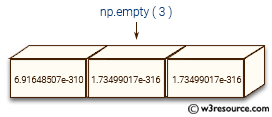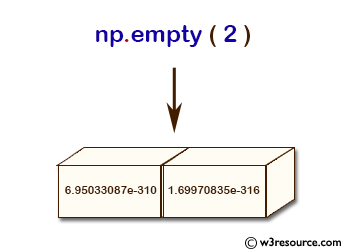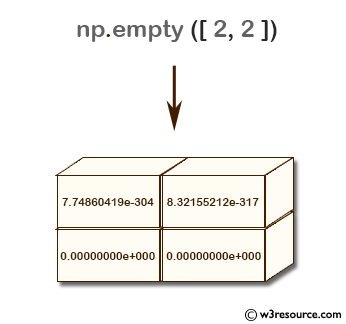# NumPy: numpy.empty() function

## numpy.empty() function

The numpy.empty() function is used to create a new array of given shape and type, without initializing entries. It is typically used for large arrays when performance is critical, and the values will be filled in later.

Syntax:

`numpy.empty(shape, dtype=float, order='C')`Parameters:

Name Description Required /
Optional
shape Shape of the empty array, e.g., (2, 3) or 2. Required
dtype Desired output data-type for the array, e.g, numpy.int8. Default is numpy.float64. optional
order Whether to store multi-dimensional data in row-major ('C' for C-style) or column-major ('F' for Fortran-style) order in memory. optional argument representing the memory layout of the array. optional

Return value:

[ndarray] Array of uninitialized (arbitrary) data of the given shape, dtype, and order. Object arrays will be initialized to None.

Example: Create empty NumPy arrays using np.empty()

``````>>> import numpy as np
>>> np.empty(2)
array([  6.95033087e-310,   1.69970835e-316])
>>> np.empty(32)
array([  6.95033087e-310,   1.65350412e-316,   6.95032869e-310,
6.95032869e-310,   6.95033051e-310,   6.95033014e-310,
6.95033165e-310,   6.95033167e-310,   6.95033163e-310,
6.95032955e-310,   6.95033162e-310,   6.95033166e-310,
6.95033160e-310,   6.95033163e-310,   6.95033162e-310,
6.95033167e-310,   6.95033167e-310,   6.95033167e-310,
6.95033167e-310,   6.95033158e-310,   6.95033160e-310,
6.95033164e-310,   6.95033162e-310,   6.95033051e-310,
6.95033161e-310,   6.95033051e-310,   6.95033013e-310,
6.95033166e-310,   6.95033161e-310,   2.97403466e+289,
7.55774284e+091,   1.31611495e+294])
>>> np.empty([2, 2])
array([[7.74860419e-304, 8.32155212e-317],
[0.00000000e+000, 0.00000000e+000]])
>>> np.empty([2, 3])
array([[  6.95033087e-310,   1.68240973e-316,   6.95032825e-310],
[  6.95032825e-310,   6.95032825e-310,   6.95032825e-310]])
``````

The above code demonstrates the use of np.empty() function in NumPy to create empty arrays of different sizes and data types. The np.empty() function creates an array without initializing its values, which means that the values of the array are undefined and may vary each time the function is called.

In the first example, an empty 1D array of size 2 is created, resulting in an array with two undefined values. The values are shown in the output, which may be different each time the function is called.
In the second example, an empty 1D array of size 32 is created, resulting in an array with 32 undefined values. The values are shown in the output, which may also vary each time the function is called.
In the third example, an empty 2D array of size (2, 2) is created, resulting in an array with four undefined values. The values are shown in the output, which may again vary each time the function is called.
In the fourth example, an empty 2D array of size (2, 3) is created, resulting in an array with six undefined values. The values are shown in the output, which may differ each time the function is called.

Pictorial Presentation:Example: Create empty NumPy arrays with specified data types using np.empty()

``````>>>import numpy as np
>>> np.empty([2, 2], dtype=int)
array([[140144669465496,        16250304],
[140144685653488, 140144685468840]])
>>> np.empty([2, 2], dtype=float)
array([[2.37015306e-316, 7.37071328e-315],
[9.06655519e-317, 2.00753892e-317]])
``````

Explanation: This code demonstrates how to use np.empty() function in NumPy to create empty arrays with specified data types. The dtype parameter in the np.empty() function can be used to specify the data type of the empty array.

In the first example, an empty 2D array of size (2, 2) is created with the specified data type int. The resulting array contains four undefined integer values. The values shown in the output are machine-dependent and may vary each time the function is called.

In the second example, an empty 2D array of size (2, 2) is created with the specified data type float. The resulting array contains four undefined floating-point values. The values shown in the output are also machine-dependent and may vary each time the function is called.

Pictorial Presentation:Example : Creating an empty array with a user-defined data type

Code:

``````import numpy as np

# Define a custom data type
dt = np.dtype([('Employee Name:', np.str_, 16), ('Age:', np.int32), ('Salary:', np.float64)])

# Create an empty array with the custom data type
employee = np.empty((2, 3), dtype=dt)

# Print the array
print(employee)
``````

Output:

```[[('', 0, 0.) ('', 0, 0.) ('', 0, 0.)]
[('', 0, 0.) ('', 0, 0.) ('', 0, 0.)]]
```

In the above example, we first define a custom data type that consists of three fields - `Employee Name` (string with length 16), Age (32-bit integer), and Salary (64-bit floating-point number). We then create an empty array with dimensions (2, 3) and data type dt. When we print the array, we see that it contains random values of the custom data type.

Python - NumPy Code Editor:

Previous: NumPy array Home
Next: empty_like()

﻿